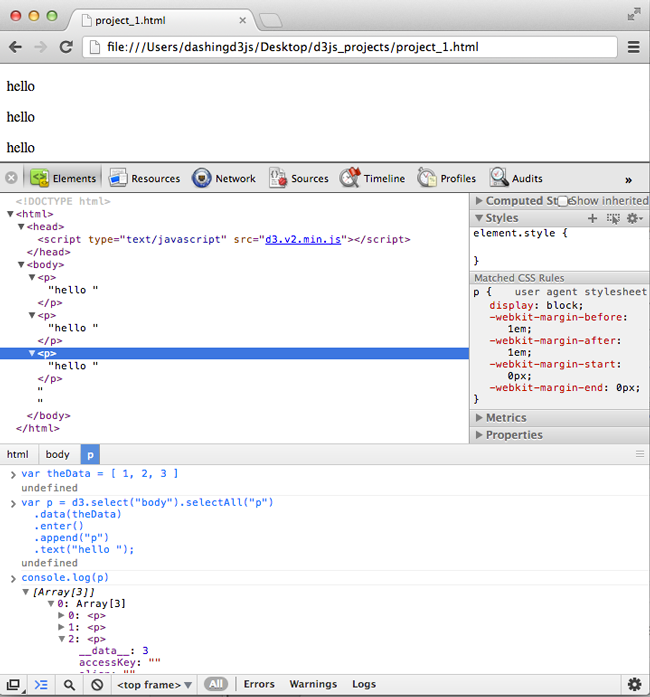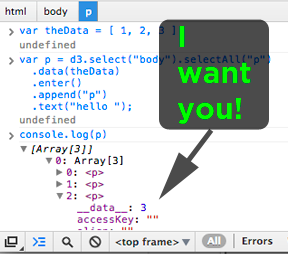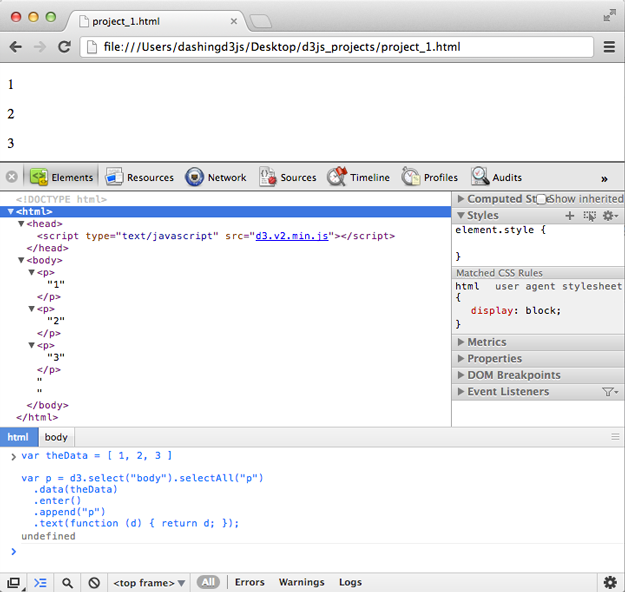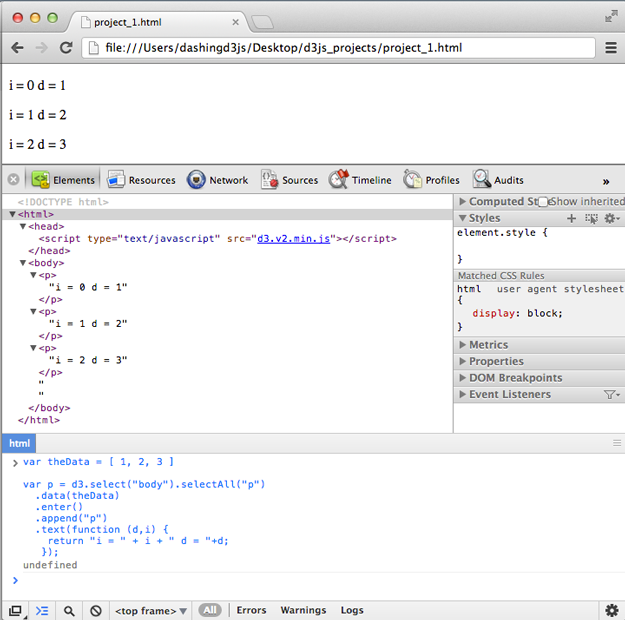# Using Data Bound to DOM Elements

### Basic Example

In this example, you will use D3.js to bind data to DOM elements of a basic webpage. Then you will use D3.js to use the data bound to DOM Elements to update the basic webpage.

In the last section, we started with a basic webpage:

```1<!DOCTYPE html>
2<html>
4    <script type="text/javascript" src="d3.v2.min.js"></script>
6  <body>
7  </body>
8</html>```

And then bound data to paragraph HTML elements through the JavaScript Console:

```1var theData = [ 1, 2, 3 ]
2
3var p = d3.select("body").selectAll("p")
4  .data(theData)
5  .enter()
6  .append("p")
7  .text("hello ");```

Which gave us this:Which is amazing - we have bound data to the DOM elements using D3.js.

However, what we really want is to get our data back on to the basic webpage.

Put another way, how do we get this value out of the DOM element?Open the Webkit Inspector to get the JavaScript Console going along side the Elements Inspector.

Type the following into the JavaScript Console:

```1var theData = [ 1, 2, 3 ]
2
3var p = d3.select("body").selectAll("p")
4  .data(theData)
5  .enter()
6  .append("p")
7  .text( function (d) { return d; } );```

Which gives us the following:As you can see - instead of three paragraphs that say "Hello", we now have three paragraphs that contain our data! Congratulations - you have used data bound to DOM elements to update the webpage using D3.js!

### D3.js Text Operator Revisited

The only part of the JavaScript code that changed from our previous example to this example is that

`1.text( "hello ");`

became:

`1.text( function (d) { return d; } );`

The selection that the .text() operator is working on in both cases is the following:

```1d3.select("body").selectAll("p")
2  .data(theData)
3  .enter()
4  .append("p")```

This selection represents three HTML <p> elements.

As we covered in the previous section, the Text Operator sets the textContent of the node to the specified value on all selected elements.

What is also true of the Text Operator is that if the value passed to it (the Text Operator) is a function, then the function is evaluated for each element in order. And the functions result is used to set each element's text context.

Which in our case means, that instead of setting "Hello" as the text value, the function goes through each element, gets the __data__ property and returns it to the Text Operator, so that it can set that result as the text content for the element.

### JavaScript Functions in D3.js Operators

We are passing a JavaScript function to the Text operator:

`1function (d) { return d; }`

If you are new to JavaScript functions, here is a brief synopsis:

```1function functionName (variableName) {
2  return variableName;
3}```

The "function" operator defines a function named "functionName" which takes a variable "variableName" and then returns the same variable.

If the functionName is missing, then it is called an anonymous function. Anonymous functions are used to make code more concise when declaring a function that will only be used in one place. In our case, the function is used only inside of the D3.js Text Operator:

`1.text( function (d) { return d; } );`

Note - our function doesn't have to be one line or just a simple return, we could so something like this:

```1function (d) {
3  var tempNumber;
4  // we add the number 2 to our input
5  tempNumber = d + addedNumber;
6  return tempNumber;
7}```

Which when put into the JavaScript Console as part of our overall D3.js functions, gives us the following:As you can see, our function has added the number "2" to our data set and updated the text of the DOM elements.

Using these functions, you can write anything you want using JavaScript to manipulate the data. This is powerful in data visualizations when you will want differentiate style and format of SVG objects based on what values the data contains.

### Variables Available inside D3.js Operators

In our example:

`1.text( function (d) { return d; } );`

You can see that the variable d is available for use in the anonymous function. This variable is provided to us by D3.js and it refers to the current __data__ attribute for the specific element being processed.

The are two other variables provided to us by D3.js - this and i.

this refers to the current DOM element being evaluated.

i refers to the index of the current HTML element being evaluated in the selected elements selection. Remember that all the data is processed at once and in sequential order. Note - i starts at 0. This means in our data set

`1var theData = [ 1, 2, 3 ]`

The data is processed in order of "1", "2" and then "3". With "1" having an i of 0, "2" having an i of 1 and "3" having an i of 2.

You can run the following in the JavaScript Console to see this demonstrated:

``` 1var theData = [ 1, 2, 3 ]
2
3var p = d3.select("body").selectAll("p")
4  .data(theData)
5  .enter()
6  .append("p")
7  .text(function (d,i) {
8    return "i = " + i + " d = "+d;
9   });```

Which gives us the following:Having the index available allows for things like alternating style and formatting.

If you found this D3 Tutorial helpful, you'll enjoy these FREE videos: